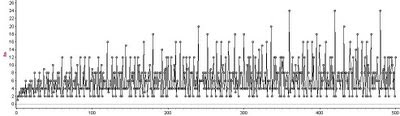## Monday, November 10, 2008

### A Bit More on Factors and Extended Multiplication TablesAs described in earlier posts, factor lattices and the extended multiplication table have a common link in an arithmetic function that we'll just call f.In the formula above, the p_i values are distinct primes. The function f tells us the number of times n appears in the extended multiplication table, and tells us the number of nodes in n's factor lattice. Note that f(1) = 1, and f(p) = 2 for every prime number p.

We can see from its formula that f(n) is multiplicative - if n and m are relatively prime, then f(nm) = f(n)f(m). This fact makes it easier to calculate f(n) values.

Other multiplicative arithmetic functions include the Euler totient function, theta(n), and the function that sums the divisors of n, sigma(n).

The graph at the top of this post shows the first 500 values of f(n).